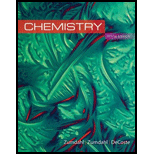# Use the postulates of the kinetic molecular theory (KMT) to explain why Boyle’s law, Charles’s law, Avogadro’s law, and Dalton’s law of partial pressures hold true for ideal gases. Use the KMT to explain the P versus n (at constant V and T) relationship and the P versus T (at constant V and n) relationship.### Chemistry

10th Edition
Steven S. Zumdahl + 2 others
Publisher: Cengage Learning
ISBN: 9781305957404

#### Solutions

Chapter
Section### Chemistry

10th Edition
Steven S. Zumdahl + 2 others
Publisher: Cengage Learning
ISBN: 9781305957404
Chapter 5, Problem 6RQ
Textbook Problem
65 views

## Use the postulates of the kinetic molecular theory (KMT) to explain why Boyle’s law, Charles’s law, Avogadro’s law, and Dalton’s law of partial pressures hold true for ideal gases. Use the KMT to explain the P versus n (at constant V and T) relationship and the P versus T (at constant V and n) relationship.

Interpretation Introduction

Interpretation: The explanation for Boyle’s law, Charles’s law, Avogadro’s law and Dalton’s law holds good for ideal gases has to be shown using Kinetic molecular theory and the explanation for relationship between P vs. n (at constant V and T) and P vs. T(at constant V and n) has to be given.

Concept Introduction:

Postulates of Kinetic theory:

1. 1. In a gas, there are a huge number of tiny particles called as molecules of similar size and weight.
2. 2. Unlike solids, the interaction between the molecules are said to be negligible.
3. 3. In a gas, the state of molecules is in random motion with different velocities from zero to infinity in all possible direction.
4. 4.  On comparison with volume of gas, the size of the molecule is negligible.
5. 5. A perfect elastic collision should take place when the molecules collide with each other.
6. 6. A pressure is exerted as the molecules collide on the walls of the container. During the impact of collision, the pressure of gas is the average force per unit area of the molecules. Higher will be the pressure, larger the number of impacts.
7. 7. Because of random motion of molecules in gas, molecules posses kinetic energy that varies as absolute temperature.

### Explanation of Solution

Explanation

Boyle’s law is one of the gas laws, states that for a known mass of gas at steady temperature, the pressure (P) is inversely proportional to its volume (V). The Boyle’s law can be expressed as,

P=(nRT)1V

Where nRT=constant

Boyle’s law: P%1/V at constant n and T.

According to kinetic molecular theory, pressure is relative to the collision frequency, which in turn is proportional to 1/V . The increase in volume will result in fewer collisions per unit area with the walls of the container and decrease in pressure.

Charles’s law states that for a given mass of gas, at constant pressure (P) and n, the volume of the gas is directly proportional to the temperature (T). The expression for Charles’s law is given as,

VαTVT=constant

Charles’s law: V%T at constant n and P.

On heating the gas to higher temperature, there is an increase in the velocities of the gas molecules and they hit the walls of the container very often and with excess force. To maintain the pressure constant, there should be an increase in the volume of the container (increasing the surface area, decreases the number of collisions per unit area, thus decreasing the pressure). Henceforth, volume and temperature are directly proportional at constant n and P.

Avogadro’s law states that for a given quantity of gas, at constant temperature and pressure, the volumes of various gases are directly proportional to their number of molecules. The expression for Avogadro’s law is given as,

VαnVn=constant

Avogadro’s law: V%n at constant P and T

When a gas is added to a container (n increases), there would be an instant increase in the number of gas particle collisions with the walls of the container. This would effect in increase in pressure in container. The container is maintained in such way that pressure is constant. To maintain a constant pressure, there should be an increase in volume of the container to reduce the collision frequency which decreases the pressure. Therefore, V is directly proportional to n at constant P and T

### Still sussing out bartleby?

Check out a sample textbook solution.

See a sample solution

#### The Solution to Your Study Problems

Bartleby provides explanations to thousands of textbook problems written by our experts, many with advanced degrees!

Get Started

Find more solutions based on key concepts
Which of these advantages do sports drinks provide over plain water? They taste good and so may lead people to ...

Nutrition: Concepts and Controversies - Standalone book (MindTap Course List)

What is the difference between a hypothesis , theory, and a law?

Horizons: Exploring the Universe (MindTap Course List)

What is genomics?

Human Heredity: Principles and Issues (MindTap Course List)

A high fountain of water is located at the center of a circular pool as shown in Figure P159. A student walks a...

Physics for Scientists and Engineers, Technology Update (No access codes included)

How does a population differ from a community?

Oceanography: An Invitation To Marine Science, Loose-leaf Versin